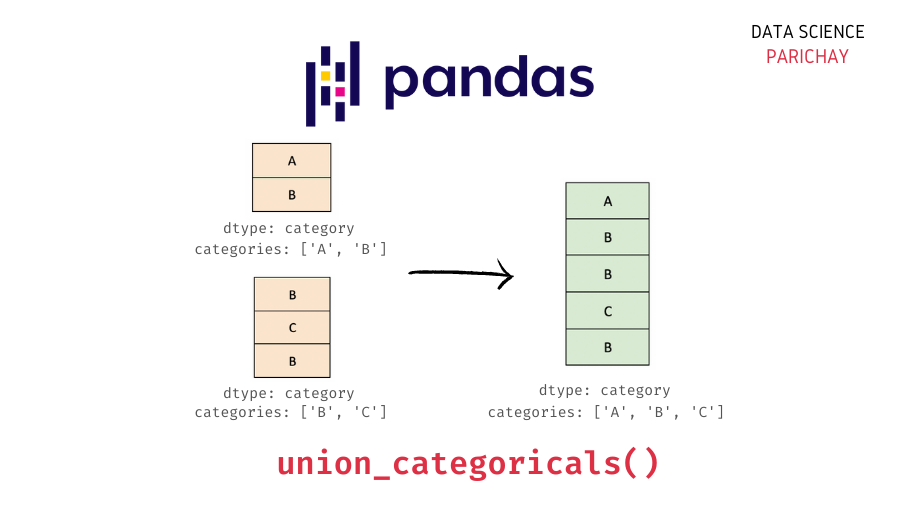# Join Category Columns with Pandas union_categoricals()

Pandas is a popular library for data manipulation in Python. It comes with a handy category dtype for categorical data. It also has useful functions to help you work with categorical data. In this tutorial, we will look at how to join two category type series in Pandas using the Pandas union_categoricals() function.

## Pandas concat() vs union_categoricals()

You can use both the Pandas `concat()` function and the `union_categoricals()` function to combine category type data. For example, both the functions give similar outcomes when combining category data having the same categories.

```import pandas as pd
from pandas.api.types import union_categoricals

# create category type pandas series
s1 = pd.Series(['a', 'b']).astype('category')
s2 = pd.Series(['b', 'a', 'a']).astype('category')

# combine the series with concat()
print(pd.concat([s1, s2]))
print("-------")
# combine the series with union_categoricals()
print(union_categoricals([s1, s2]))```

Output:

```0    a
1    b
0    b
1    a
2    a
dtype: category
Categories (2, object): ['a', 'b']
-------
['a', 'b', 'b', 'a', 'a']
Categories (2, object): ['a', 'b']```

Here, we combine two category type Pandas series, s1 and s2, both having the same categories – “a” and “b”.

Note that, with `concat()` we get a Pandas series whereas with `union_categoricals()` we get a Pandas categorical array. You can see that both the methods result in a category type outcome with the same unique categories.

But, if you use the Pandas `concat()` function to join categorical series with different category values, the resulting series is not of `category` type.

```# create category type pandas series
s1 = pd.Series(['a', 'b']).astype('category')
s2 = pd.Series(['b', 'd', 'c']).astype('category')

# combine the series with concat()
print(pd.concat([s1, s2]))```

Output:

```0    a
1    b
0    b
1    d
2    c
dtype: object```

Let’s now join the two series with different category values using the Pandas `union_categoricals()` function.

📚 Data Science Programs By Skill Level

Introductory

Intermediate ⭐⭐⭐

🔎 Find Data Science Programs 👨‍💻 111,889 already enrolled

Disclaimer: Data Science Parichay is reader supported. When you purchase a course through a link on this site, we may earn a small commission at no additional cost to you. Earned commissions help support this website and its team of writers.

```# create category type pandas series
s1 = pd.Series(['a', 'b']).astype('category')
s2 = pd.Series(['b', 'd', 'c']).astype('category')

# combine the series with union_categoricals()
print(union_categoricals([s1, s2]))```

Output:

```['a', 'b', 'b', 'd', 'c']
Categories (4, object): ['a', 'b', 'c', 'd']```

You can see that the outcome is categorical with categories from both the joined series.

Thus, a key advantage of using `union_categories()` is that you can use it to join categorical data with different categories into a category type outcome.

### Pandas `union_categoricals()` on ordered categorical data

You can also use the `union_categoricals()` on ordered categorical data.

```# create category type pandas series
s1 = pd.Series(['a', 'b']).astype('category')
s1 = s1.cat.set_categories(['a', 'b'], ordered=True)
s2 = pd.Series(['b', 'a', 'b']).astype('category')
s2 = s2.cat.set_categories(['a', 'b'], ordered=True)

# combine the series with union_categoricals()
print(union_categoricals([s1, s2]))```

Output:

```['a', 'b', 'b', 'a', 'b']
Categories (2, object): ['a' < 'b']```

Here, we combine two series having the same categories and the same category order. You can see that the result is also an ordered categorical having the same category order.

Let’s see what happens if you combine two series with different categories or orders.

```# create category type pandas series
s1 = pd.Series(['a', 'b']).astype('category')
s1 = s1.cat.set_categories(['a', 'b'], ordered=True)
s2 = pd.Series(['b', 'a', 'c']).astype('category')
s2 = s2.cat.set_categories(['a', 'b', 'c'], ordered=True)

# combine the series with union_categoricals()
print(union_categoricals([s1, s2]))```

Output:

```---------------------------------------------------------------------------
TypeError                                 Traceback (most recent call last)
Input In , in <module>
5 s2 = s2.cat.set_categories(['a', 'b', 'c'], ordered=True)
7 # combine the series with union_categoricals()
----> 8 print(union_categoricals([s1, s2]))
...
TypeError: to union ordered Categoricals, all categories must be the same```

We get an error since to combine ordered categoricals using `union_categoricals()`, all categories (and their relative order) must be the same.

To join ordered categoricals with different categories or orders, you can use the `ignore_order=True` argument.

```# create category type pandas series
s1 = pd.Series(['a', 'b']).astype('category')
s1 = s1.cat.set_categories(['a', 'b'], ordered=True)
s2 = pd.Series(['b', 'a', 'c']).astype('category')
s2 = s2.cat.set_categories(['a', 'b', 'c'], ordered=True)

# combine the series with union_categoricals()
print(union_categoricals([s1, s2], ignore_order=True))```

Output:

```['a', 'b', 'b', 'a', 'c']
Categories (3, object): ['a', 'b', 'c']```

We didn’t get an error this time. Notice that the resulting categorical is unordered.

You might also be interested in –

•